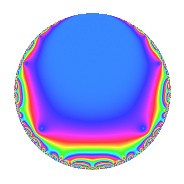# Properties

 Label 99.2.a.dLevel 99 Weight 2 Character orbit 99.a Self dual Yes Analytic conductor 0.791 Analytic rank 0 Dimension 1 CM No Inner twists 1

# Learn more about

## Newspace parameters

 Level: $$N$$ = $$99 = 3^{2} \cdot 11$$ Weight: $$k$$ = $$2$$ Character orbit: $$[\chi]$$ = 99.a (trivial)

## Newform invariants

 Self dual: Yes Analytic conductor: $$0.790518980011$$ Analytic rank: $$0$$ Dimension: $$1$$ Coefficient field: $$\mathbb{Q}$$ Coefficient ring: $$\mathbb{Z}$$ Coefficient ring index: $$1$$ Fricke sign: $$-1$$ Sato-Tate group: $\mathrm{SU}(2)$

## $q$-expansion

 $$f(q)$$ $$=$$ $$q$$ $$\mathstrut +\mathstrut 2q^{2}$$ $$\mathstrut +\mathstrut 2q^{4}$$ $$\mathstrut -\mathstrut q^{5}$$ $$\mathstrut -\mathstrut 2q^{7}$$ $$\mathstrut +\mathstrut O(q^{10})$$ $$q$$ $$\mathstrut +\mathstrut 2q^{2}$$ $$\mathstrut +\mathstrut 2q^{4}$$ $$\mathstrut -\mathstrut q^{5}$$ $$\mathstrut -\mathstrut 2q^{7}$$ $$\mathstrut -\mathstrut 2q^{10}$$ $$\mathstrut -\mathstrut q^{11}$$ $$\mathstrut +\mathstrut 4q^{13}$$ $$\mathstrut -\mathstrut 4q^{14}$$ $$\mathstrut -\mathstrut 4q^{16}$$ $$\mathstrut +\mathstrut 2q^{17}$$ $$\mathstrut -\mathstrut 2q^{20}$$ $$\mathstrut -\mathstrut 2q^{22}$$ $$\mathstrut +\mathstrut q^{23}$$ $$\mathstrut -\mathstrut 4q^{25}$$ $$\mathstrut +\mathstrut 8q^{26}$$ $$\mathstrut -\mathstrut 4q^{28}$$ $$\mathstrut +\mathstrut 7q^{31}$$ $$\mathstrut -\mathstrut 8q^{32}$$ $$\mathstrut +\mathstrut 4q^{34}$$ $$\mathstrut +\mathstrut 2q^{35}$$ $$\mathstrut +\mathstrut 3q^{37}$$ $$\mathstrut +\mathstrut 8q^{41}$$ $$\mathstrut -\mathstrut 6q^{43}$$ $$\mathstrut -\mathstrut 2q^{44}$$ $$\mathstrut +\mathstrut 2q^{46}$$ $$\mathstrut -\mathstrut 8q^{47}$$ $$\mathstrut -\mathstrut 3q^{49}$$ $$\mathstrut -\mathstrut 8q^{50}$$ $$\mathstrut +\mathstrut 8q^{52}$$ $$\mathstrut +\mathstrut 6q^{53}$$ $$\mathstrut +\mathstrut q^{55}$$ $$\mathstrut -\mathstrut 5q^{59}$$ $$\mathstrut +\mathstrut 12q^{61}$$ $$\mathstrut +\mathstrut 14q^{62}$$ $$\mathstrut -\mathstrut 8q^{64}$$ $$\mathstrut -\mathstrut 4q^{65}$$ $$\mathstrut -\mathstrut 7q^{67}$$ $$\mathstrut +\mathstrut 4q^{68}$$ $$\mathstrut +\mathstrut 4q^{70}$$ $$\mathstrut +\mathstrut 3q^{71}$$ $$\mathstrut +\mathstrut 4q^{73}$$ $$\mathstrut +\mathstrut 6q^{74}$$ $$\mathstrut +\mathstrut 2q^{77}$$ $$\mathstrut -\mathstrut 10q^{79}$$ $$\mathstrut +\mathstrut 4q^{80}$$ $$\mathstrut +\mathstrut 16q^{82}$$ $$\mathstrut +\mathstrut 6q^{83}$$ $$\mathstrut -\mathstrut 2q^{85}$$ $$\mathstrut -\mathstrut 12q^{86}$$ $$\mathstrut -\mathstrut 15q^{89}$$ $$\mathstrut -\mathstrut 8q^{91}$$ $$\mathstrut +\mathstrut 2q^{92}$$ $$\mathstrut -\mathstrut 16q^{94}$$ $$\mathstrut -\mathstrut 7q^{97}$$ $$\mathstrut -\mathstrut 6q^{98}$$ $$\mathstrut +\mathstrut O(q^{100})$$

## Embeddings

For each embedding $$\iota_m$$ of the coefficient field, the values $$\iota_m(a_n)$$ are shown below.

For more information on an embedded modular form you can click on its label.

Label $$\iota_m(\nu)$$ $$a_{2}$$ $$a_{3}$$ $$a_{4}$$ $$a_{5}$$ $$a_{6}$$ $$a_{7}$$ $$a_{8}$$ $$a_{9}$$ $$a_{10}$$
1.1
 0
2.00000 0 2.00000 −1.00000 0 −2.00000 0 0 −2.00000
 $$n$$: e.g. 2-40 or 990-1000 Significant digits: Format: Complex embeddings Normalized embeddings Satake parameters Satake angles

## Inner twists

This newform does not admit any (nontrivial) inner twists.

## Atkin-Lehner signs

$$p$$ Sign
$$3$$ $$-1$$
$$11$$ $$1$$

## Hecke kernels

This newform can be constructed as the intersection of the kernels of the following linear operators acting on $$S_{2}^{\mathrm{new}}(\Gamma_0(99))$$:

 $$T_{2}$$ $$\mathstrut -\mathstrut 2$$ $$T_{5}$$ $$\mathstrut +\mathstrut 1$$